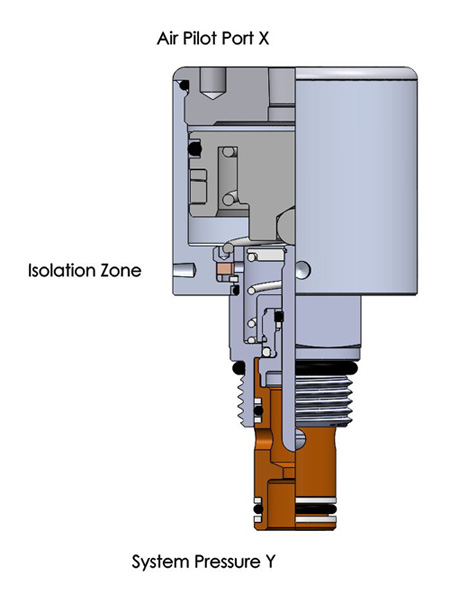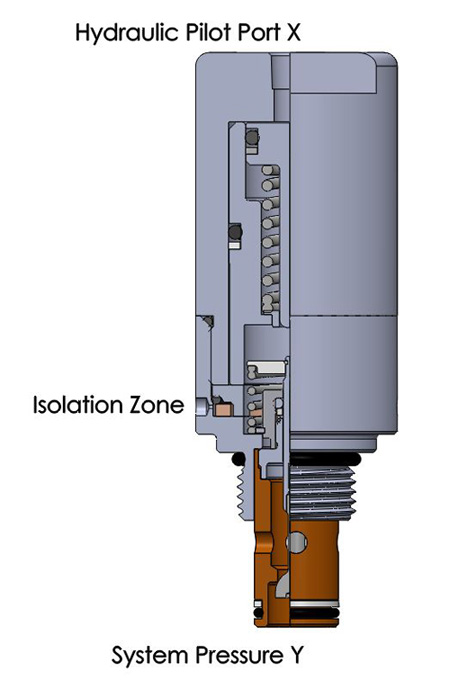# Pilot Ratio Calculator

• Air Pilot
• PSI bar
• PSI bar
• =(Y*1.1)/(X*.9)
Round up to standard available pilot ratio.• Hydraulic Pilot
• PSI bar
• PSI bar
• =(Y*1.1)/((X*.9)-100 PSI (6.9 BAR))
Round up to standard available pilot ratio.How do I know how much pilot pressure is required to shift a valve?

To calculate the correct pilot ratio valve, use the following equations. These equations take into consideration the spring forces in the valve; however, this is under ideal conditions, they do not consider any effects of temperature of the oil or any backpressure in the system.

Air Pilot Valve:

1. Determine MINIMUM possible pilot pressure. Multiply available pilot pressure by .9 = PILOT (X)
2. Determine the MAXIMUM possible system pressure. Multiply system pressure by 1.1 = SYSTEM (Y)
3. Divide SYSTEM (Y) by PILOT (X) = PILOT TO SYSTEM RATIO
(Y*1.1)/(X*.9) = AIR PILOT RATIO
4. Round up to standard available ratio

Hydraulic Pilot Valve:

1. Determine MINIMUM possible pilot pressure. Multiply the pilot pressure by .9 then subtract 100 PSI = PILOT (X)
2. Determine the MAXIMUM possible system pressure. Multiply system pressure by 1.1 = SYSTEM (Y)
3. Divide SYSTEM (Y) by PILOT (X) = PILOT TO SYSTEM RATIO
(Y*1.1)/((X*.9)-100 PSI (6.9 BAR)) = HYDRAULIC PILOT RATIO
4. Round up to standard available ratio